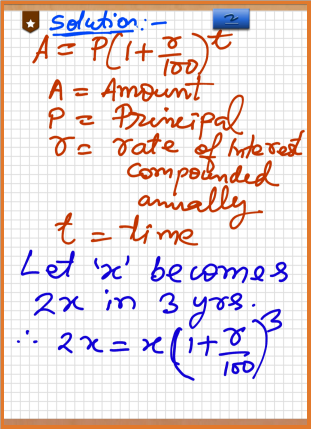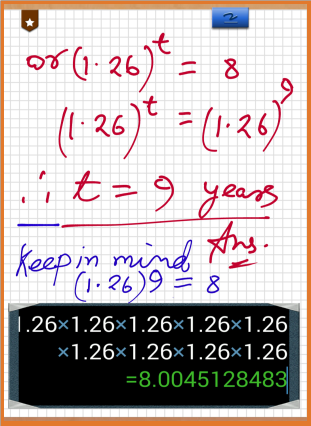Mar 24, 2015

# Basic of Calender Learning for Grade 2 | Mathematics

The concept of the calender originated from solar year. The earth completes its orbit about the sun in 365 days 5 hr 48 min 46 sec.This is called solar year.

Total Number of months in a year = 12
Total Number of days in a year = 365
Total Number of days in a leap year = 366

If any year is divisible by 4 then the year is called a leap year. For example: 2008, 2012, 2016 etc are the leap years.

To determine the number of days of any mount we count on our hand's back as shown in second diagram.
Total Number of months of 31 days in a year = 7
Total Number of months of 30 days in a year = 4
Total Number of months of 28 days or of 29 days in a year = 1

Mar 22, 2015

# Unitary Method in Simple words - Learn Maths Basics

Unitary means "of one" . Mathematically it means "1". To know this in an easy way, I am writing few examples.

Question 1: If the cost of one pen is Rs 2 . Find the cost of 25 Pens
Question 2: If the cost of 50 pencils is Rs 500. oo . Find the cost of One pencil .

I am modifying the above Sum a little bit .

Question 3: If the cost of 50 pencils is Rs 500.00 . Find the cost of 12 pencil .Now the basic rule is :

For question 1. If we know the cost or other parameter of one thing , then to know the cost of many thing we will do multiplication .
Here we know the cost of 1 pen . Therefore the cost of 25 pen will be MORE than the cost of 1 one pen.
Here we realize for more value . Therefore we will multiply.
Also watch the image cost of ONE to cost of MANY means MULTIPLY.
Solution 1:
∵ the cost of 1 pen is Rs. 2
∴ the cost of 25 pens is 2x25 [we realize for more value.]
= Rs. 50

For question 2. And if we know the cost of many things or other Parameter , then to know the cost of one thing we will do division.
Here we know the cost of many pencils and we want to know the cost of 1 pencil.
We realise that the cost of 1 pencil will less than the cost of 50 pencils.
Therefore we realise for less value. Therefore we will divide.
Solution 2:
∵ the cost of 50 pencils is Rs. 500
∴ the cost of 1 pencil is 500/25    [we realize for less value. Therefore divide]
= Rs. 20

### 3rd sum is the combination of two sums. In this sum we will realise both division and multiplication.

Solution 3:
∵ the cost of 50 pencils is Rs. 500
∴ the cost of 1 pencil is 500/25 [we realize for less value. Therefore divide]
= Rs. 20
∴ the cost of 12 pencils is 20x12 [we realize for more value.Therefore multiply.]
= Rs. 240

Now watch this video.

Mar 17, 2015

# Speed Distance Time Math Class 8 Part 1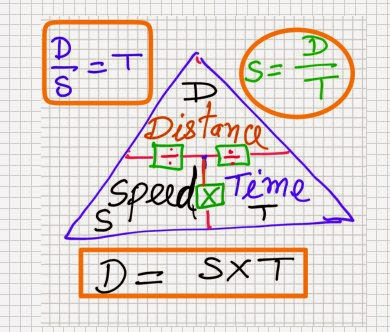The most important thing about this chapter is that the sums are very easy and can be solved by most of the students if the formula is known to him. Also it is more important to keep in memory the formula of speed, distance and time. The third most important point to note is the unit of speed given in the sum and units of distance and time. It is here the student's minds are confused. Be clear about km/hr and m/sec and mile/hr.

For converting km/hr to m/s multiply by as it can be noticed in the above image. And for converting m/s to km/hr multiply by 18/5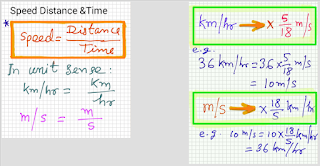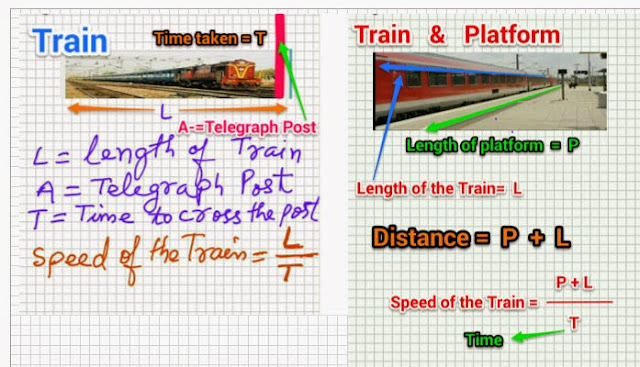Sometime it is written as :
60 kmph = it means 60 km/hr
60 mph = it means 60 m/hr or 60 mile/hr

Mar 11, 2015

# The wizard of oz | The Yellow Brick Road | English Literature

Question 1:What does the scarecrow want most of all and why?
Answer:The scarecrow wanted to get some brain. The scarecrow wants some brain because it's head was stuffed with straw.

Question 2 : what has happened to the Tin wood man? What does he feel he need?
Answer : Tin woodman was a normal person who was a wood cutter. He loved and was punished by an old woman .The old woman asked a wicked witch to bewitch the woodcutter. The wood cutter was bewitched and he met with accidents at work. His head and body was replaced with tin head arm and legs. At the end he became a tin man with no brain and heart. The tin woodman felt that he needs a heart.

Question 3 : During the journey,what made the Tin wood man unhappy?
Answer : The tin woodman was unhappy because he stepped upon a beetle that was crawling along the road.

Question 4 : Why was the Tin Woodman careful when he walked?How did he explain his behaviour?
Answer : The Tin wood man killed a beetle and he was unhappy. So he walked carefully with his eyes on the road. The Tin Woodman knew very carefully that he had no heart, and therefore he took great care never to be cruel or unkind to others.

Question 5 : Dorothy and her companion.
travelled along the "Yellow Brick road". What does the author mean by this description?
Answer : The "Yellow Brick Road" through which Dorothy and her companions were travelling led them to a place which can solve their problems by fulfilling their wishes.

Mar 5, 2015

# A train left New Delhi for Chennai at 10 AM | with a speed of 70 km/hr | Another Train also left New Delhi for Chennai | at 11 AM with a speed of 90 km / hr | At what time 2nd Train will overtake the first train |The question is again rewritten here:

Question : A train left  New Delhi for Chennai at 10 AM  with a speed of 70 km/hr . Another Train also left New Delhi for Chennai  at 11 AM with a speed of 90 km / hr   .  At what time 2nd Train will overtake the first train .

Solution: The main point of understanding this sum is that the distance where the 2nd train will overtake the first one will be same . Also the time taken by the first trains will be 1 hr more than the 2nd trains.

Also remember that the slower train will take more time than the faster train.Therefor we can create in one line .
90t = 70 (t+1) <br />
where t = time taken by the 2nd trains .
* See solution in the image also.
the distances are equal .
90t-70t=20t
:. t=70/20
:. t=3.5 Hrs
Therefor 2nd trains will overtake first one at (11 + 3.5) Hrs .

Mar 4, 2015

# A sum of Money becomes double in 3 years | At a compound rate of interest | What is rate of Interest | In how many times it will become 8 times

Sometimes this type of sum are given by the teaches in which logarithm cannot be used at grade or class 8 level. Teacher want to puzzle students so that more students join to their private coaching. You need not to worry . We are here to help the needy students. Let first reed the Sum .

This sum will be shown by two method. first one is preferable for students of class 8 to class 10

Question:- A sum of money becomes double in 3 years at a compound rate of interest. Howmany years It will be its 8 times at the same rate. Find the compound rate of interest and the time in which it becomes its 8 times.

1st method

solution: Let 'x' becomes 2x in 3 years. We know that : A=P(1+r/100)t A=Amount
P= Principal
r=compound rate of interest
t=time
∵ 2x=x (1+r/100)3
∴ 2 = (1+ r/100)3
Now 8x=x(1+r/100)t
8 = (1+r/100)t
Or 2 3= (1+ r/100)t
or [(1+ r/100)3]3=(1+r/100)t
or (1+ r/100)3x3=(1+r/100)t
(1+ r/100)9=(1+r/100)t
Now the base (1+ r/100) is same at both side.
Therefore, t = 9 years. ANS.

2nd method

∵ 2x=x (1+r/100)3
∴ 1+ r/100=(2)(1/3)
∴ 1+r/100=1.2599
∴ r=26
Now 8x=x(1+26/100)^t
or 8=(1.26)^t
or (1.26)^9=(1.26)^t
Therefore, t=9 years. ANS.